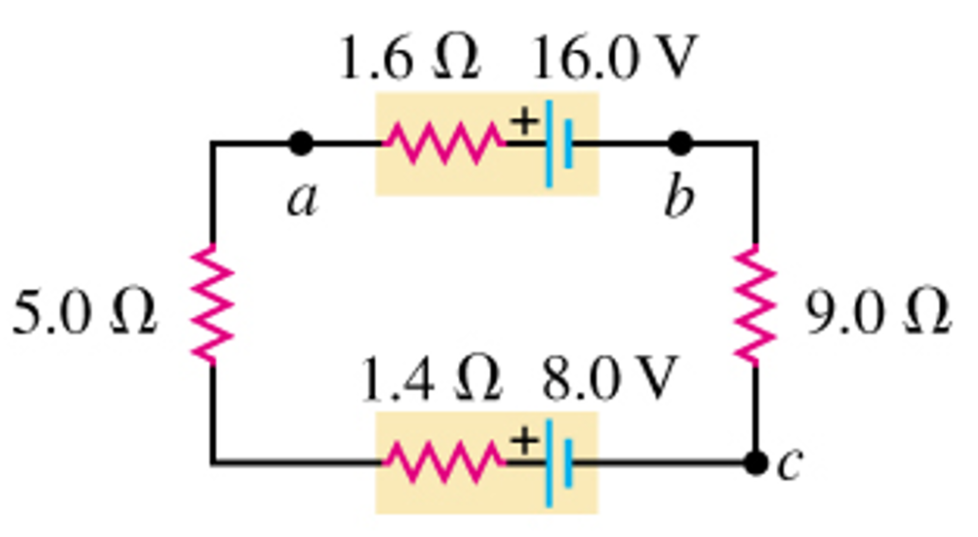# Problem: Consider the circuit of the figure (Figure 1) .(a) What is the total rate at which electrical energy is dissipated in the 5.0-Ω resistor? Express your answer using two significant figures.(b) What is the total rate at which electrical energy is dissipated in the 9.0-Ω resistor? Express your answer using two significant figures.(c) What is the power output of the 16.0-V battery? Express your answer using two significant figures.(d) At what rate is electrical energy being converted to other forms in the 8.0-V battery? Express your answer using two significant figures.

###### FREE Expert Solution

For resistors in series, the equivalent resistance is given by:

$\overline{){{\mathbf{R}}}_{\mathbf{e}\mathbf{q}}{\mathbf{=}}{{\mathbf{R}}}_{{\mathbf{1}}}{\mathbf{+}}{{\mathbf{R}}}_{{\mathbf{2}}}{\mathbf{+}}{\mathbf{.}}{\mathbf{.}}{\mathbf{.}}{\mathbf{+}}{{\mathbf{R}}}_{{\mathbf{n}}}}$

Current:

$\overline{){\mathbf{i}}{\mathbf{=}}\frac{\mathbf{\epsilon }}{{\mathbf{R}}_{\mathbf{e}\mathbf{q}}}}$

Power:

$\overline{){\mathbf{P}}{\mathbf{=}}{{\mathbf{i}}}^{{\mathbf{2}}}{\mathbf{R}}}$

or

P = iV

Req = 9 + 1.6 + 5 + 1.4 = 17Ω

i = ε/Req = ΣV/Req = (16.0 - 8.0)/17 = 0.47A

96% (249 ratings)###### Problem Details

Consider the circuit of the figure (Figure 1) .

(a) What is the total rate at which electrical energy is dissipated in the 5.0-Ω resistor? Express your answer using two significant figures.
(b) What is the total rate at which electrical energy is dissipated in the 9.0-Ω resistor? Express your answer using two significant figures.
(c) What is the power output of the 16.0-V battery? Express your answer using two significant figures.
(d) At what rate is electrical energy being converted to other forms in the 8.0-V battery? Express your answer using two significant figures.